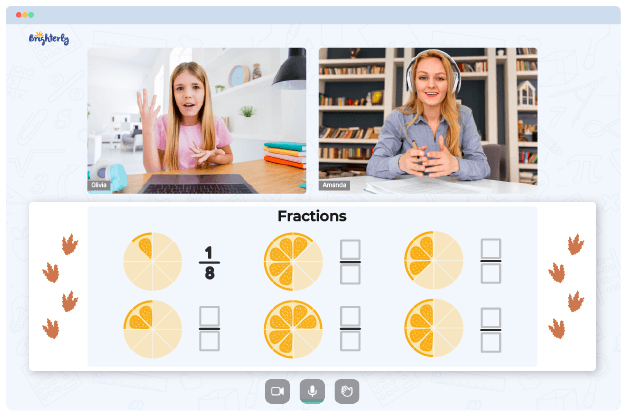# Fractions, Decimals And Percents Worksheets

Students will master fundamental arithmetic skills by practicing with the worksheets on fractions, decimals, and percents. These three concepts are essential in everyday life and throughout kids’ mathematics learning. Considering the importance of these mathematical concepts, kids must master them with quality resources like Brighterly’s worksheets.

## Concepts of Decimal, Percent, and Fraction

In mathematics, a fraction is a numeric expression that represents a part of some more significant number. Decimal describes numbers representing a whole’s fractions, such as tenths, hundredths, etc. Percentages are fractions expressed as one-hundredth of a unit.

## Free Fraction Decimal Percent Worksheet

A free fraction to decimal to percent worksheet is designed to deepen kids’ familiarity with fractions, decimals, and percentages. With worksheets, students learn to think in new ways by using various strategies to answer the problems.

Kids will encounter questions where they will have to solve problems that require them to compare and arrange a series of fractional and decimal values.

Math for Kids

Is Your Child Struggling With Math?
1:1 Online Math TutoringThe fraction, decimal, and percent worksheets for graders are excellent for helping pupils master fundamental and more complex topics. These worksheets have questions ranging from easy to moderate, and then to challenging ones.

The differing difficulty levels of worksheets allow for stepwise learning. Students will start practice from the basics before moving on to more advanced questions. The more challenging questions in the fraction decimal percent conversion worksheet will help students who are already comfortable with the basics of working with fractions and decimals.

## Printable Fraction Decimal Percent Worksheet PDF### Fractions, Decimals And Percents Worksheets PDF

Fraction Decimal Percent Worksheet### Fractions, Decimals And Percents Worksheets PDF

Fraction Decimal Percent Conversion Worksheet### Fractions, Decimals And Percents Worksheets PDF

Fraction Decimal Percent Worksheet PDF### Fractions, Decimals And Percents Worksheets PDF

Fraction To Decimal To Percent Worksheet

Having a printable version of the fraction decimal percent worksheet is all you need to help your kids learn independently. Also, it encourages repetitive learning, which brings about mastery.

### More Percents Worksheets

Troubles with Fractions?• Start studying with an online tutor.

Is your child finding it challenging to grasp the fundamentals of fractions? An online tutor could be the answer.